# Disclaimer: The following material is being kept online for archival purposes.

## Although accurate at the time of publication, it is no longer being updated. The page may contain broken links or outdated information, and parts may not function in current web browsers.

 Site Map Math Index Glossary Timeline Questions & Answers Lesson Plans (Opt) Rotation of Axes #M-12. The Tangent

# (M-12) Trigonometry Proficiency Drill

 Sections (M 6-11) gave you some principles of trigonometry. This section can provide you with practice in applying them. In some cases solutions are given, but do not peek at them until you have made a determined effort to solve them on your own
 We have tried to avoid repetitive excercises: each set is different. Do them all--do not leave any out! It is assumed that you have available a calculator which can derive sines and cosines, and also has functions sin-1 and cos-1 which given sinA or cosA can find the angle A, in the range 0 to 180 degrees.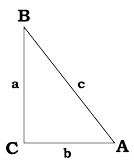A triangle ABC has a right angle C and two sharp (or "acute") angles A and B. The triangle's sides AC and BC on both sides of the right angle C are given as: (a) AC = 3     BC = 4 (b) AC = 5     BC = 12 (c) AC = 8     BC = 15 In each case, use the theorem of Pythagoras to find the third side and then find the sine and cosine of angles A and B. You are traveling uphill on a road and see a sign telling you it has a 5% slope, i.e. rising 5 meters for every 100 meters of road. What is the angle between the road and the horizontal direction?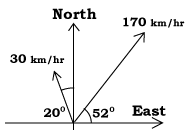An airplane is flying at 170 km/s towards the north-east, in a direction making an angle of 52° with the eastward direction. The wind is blowing at 30 km/s towards the north west, making an angle 20° with the northward direction. What is the actual "ground speed" of the airplane, and what is the angle A between the airplane's actual path and the eastward direction? (The solution below uses vector components, as derived the section on vectors: read it only after you have tried to work out the problem yourself. Teachers in class may substitute different numbers and directions) Let us denote the velocity vector of the airplane relative to the air as V, that of the wind relative to the ground as W, and the velocity of the airplane relative to the ground U=V+W, where the addition is one of vectors. Draw a diagram with the given speeds and angles labeled appropriately. To perform the actual addition each vector must be resolved into its components. We get Vx = 170 cos(52°) = 104.6    Vy = 170 sin(52°) = 133.96 Wx = –30 sin(20°) = -10.26     Wy = 30 cos(20°) = 28.19 Add: Ux = 94.4     Uy = 162.15     From Pythagoras, since   U2 = Ux2 + Uy2,             U= 187.63 km/hr     Therefore cos A = Ux /U = 0.503125 Using the cos–1 button A = 59.8°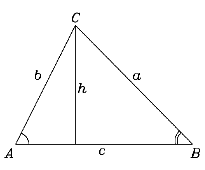In a triangle ABC, we name the angles (A,B,C) according to their corners ("vertices," singular "vertex"), and name the sides (a,b,c), so that side a is facing angle A, b faces angle B, and c faces angle C. Prove the "law of sines" sinA/a = sinB/b Hint: From C draw a line CD perpendicular to side c. The line CD is a "height" of the triangle and will therefore be denoted by the letter h. Use h in your proof. Before attempting the next problem, note two points:
 If in the above proof we had used a perpendicular "height" from A or B, rather than from C, we would have got a relation like sinA/a = sinC/c Therefore the "law of sines" is completely symmetric: sinA/a = sinB/b = sinC/c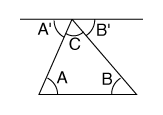The sum of angles in a triangle is always 180 degrees. A rigorous proof takes some work, but the statement can be made plausible by the following argument. In the triangle ABC, draw a line through point C that is parallel to AB. This creates two additional angles, A' and B'. The three angles (A',C,B') add up to 180 degrees, because they are adjacent to each other and backed off against a straight line. However, by the properties of parallel lines, the angles (A,A') are equal, as are (B,B'). Therefore (A,C,B) also add up to 180 degrees.
 The problem: In the triangle ABC, the line AB is along a straight riverbank. We measure the distance c = AB as 118 meters, and angles A and B are 63° and 55° . What is the distance b = AC? Don't read any further until you have tried to solve it. Teachers in class may substitute different numbers and directions.
 Because the sum of all angles is 180 degrees, the angle C must equal 62°. Then by the law of sines 118/sin(62°) = b/sin(55°) Multiply both sides by sin(55) to obtain the length b = AC. Further question: what is the perpendicular distance from C to the line c = AB? (hint: it equals the height h in the derivation of problem (4).)
 (after section M-10) Find the sine and cosine of (1) 145°         (2) 210°         (3) 300°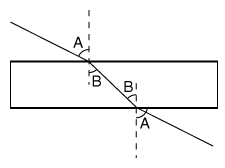(a) When a beam of light hits the surface of a flat piece of glass, it is generally bent by some angle. Draw a line perpendicular to the point on the surface where the beam enters. Then if the beam reaches the surface along a path making an angle A with the surface, it continues inside the glass with an angle making an angle B, where sin B = (sin A)/n The number n ("refractive index") is a property of the glass and is larger than one. The problem: given values of A=0, 20, 40, 60, and 80 degrees, and n = 1.45, what is B in each case? (b) the same rule holds when the light leaves the glass, except that now the angle B (inside the glass) is given and the angle A (in air) must be found. We then use
 sin A = n sinB If this formula somehow fails, the beam cannot leave the glass but is reflected from the boundary surface back into the glass, as from a mirror ("total internal reflection") The problem:Given B = 0, 20, 90, 60, 80 --what are the angles A? Does any of these beams fail to leave the glass? (By the way: the reason a prism splits light into colors is that the value of n depends slightly on color of the light--or more accurately, on its wavelength).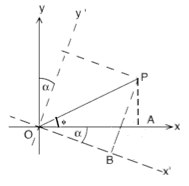In a set of cartesian coordinates the point P has coordinates (x,y), and as shown in the drawing, x = OA, y = PA. Comverting to polar cordinates (r, φ) r2 = x2 + y2 x = r cos φ y = r sin φ A second system with the same origin O has axes (x',y') formed by a clockwise rotation of the (x,y) axes by an angle α. Problem: (with help of the figure drawn here, try solving by yourself before reading the solution below). In the drawn figure     x' = OB y'= PB x' = r cos (φ + α) y' = r sin (φ + α) Using the formula for sums of angles and rearranging x' = r [cosφ cosα - sinφ sinα]  =  (r cosφ) cosα - (r sinφ) sinα y' = r [cosφ sinα + sinφ cosα]  =  (r cosφ) sinα + (r sinφ) cosα Converting back to rectangular coordinates x' = x cosα - y sinα y' = x sinα + y scosα These are the basic formulas for converting rectangular coordinates (x,y) to a system (x',y') rotated by an angle φ around the same origin, How long is the line of latitude L if the Earth is a sphere and the equator-to-pole distance is 10,000 km? How long is one degree of longitude at latitude L?     Does the formula also hold for southern latitude? (a) If cos X = 2 sinX, what is sin X? What is the angle X? (b) Is this the only solution?     Hints:(a) Use cos2X = 4 sin2X (b) Since we work with an equation that only involves squares, any solution of it only stipulates sin2X and cos2X. However, the original condition also requires that cos X and sin X have the same algebraic sign.

Author and Curator:   Dr. David P. Stern
Mail to Dr.Stern:   stargaze("at" symbol)phy6.org .

Last updated 25 November 2001